GeeksforGeeks App
Open AppBrowser
Continue

# SQL Server Mathematical functions (SQRT, PI, SQUARE, ROUND, CEILING & FLOOR)

Mathematical functions are present in SQL server which can be used to perform mathematical calculations. Some commonly used mathematical functions are given below:
1. SQRT():
SQRT() function is the most commonly used function. It takes any numeric values and returns the square root value of that number.

Syntax:

`SELECT SQRT(..value..)`

Example: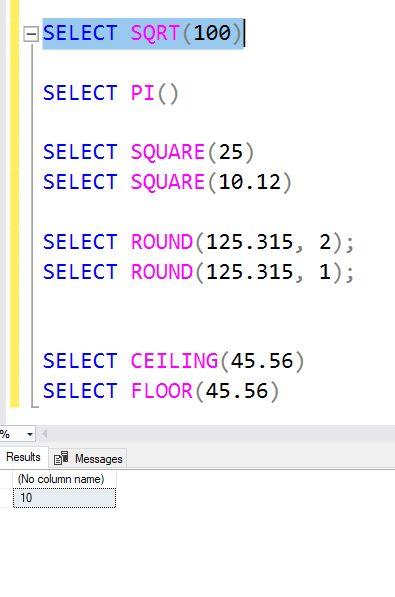2. PI(): There are calculations which require use of pi. Using pi() function, value of PI can be used anywhere in the query.

Syntax:

`SELECT PI()`

Example: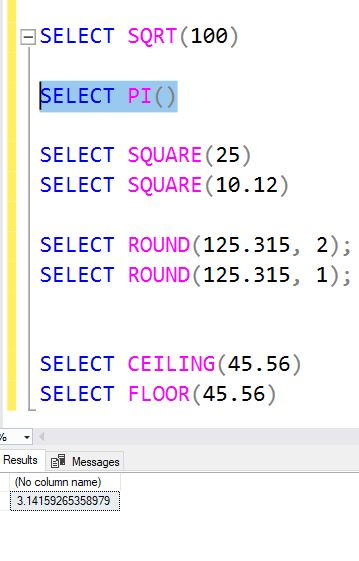3. SQUARE(): SQUARE() function is used to find the square of any number.

Syntax:

`SELECT SQUARE(..value..)`

Example: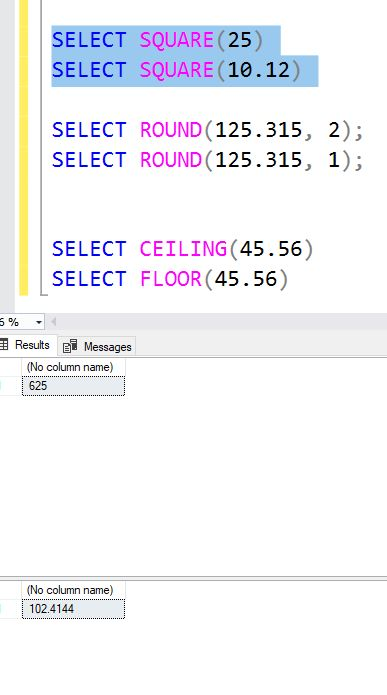4. ROUND(): ROUND() function is used to round a value to the nearest specified decimal place.

Syntax:

`SELECT ROUND(..value.., number_of_decimal_places)`

Example: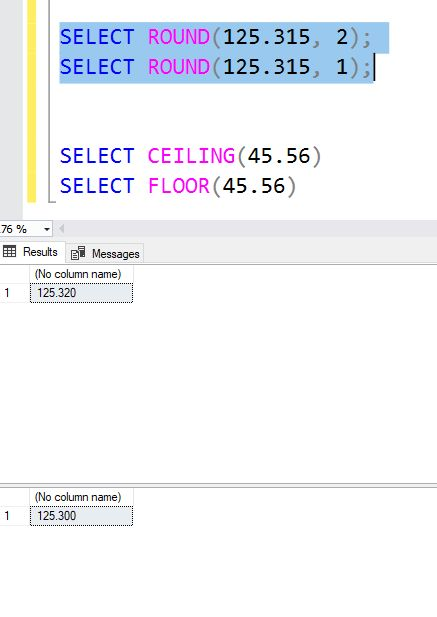5. CEILING() and FLOOR()
CEILING(): CEILING() function is used to find the next highest value (integer).

Syntax:

`SELECT CEILING(..value..)`

FLOOR(): FLOOR() function returns the next lowest value (integer).
Syntax:

`SELECT FLOOR(..value..)`

Example: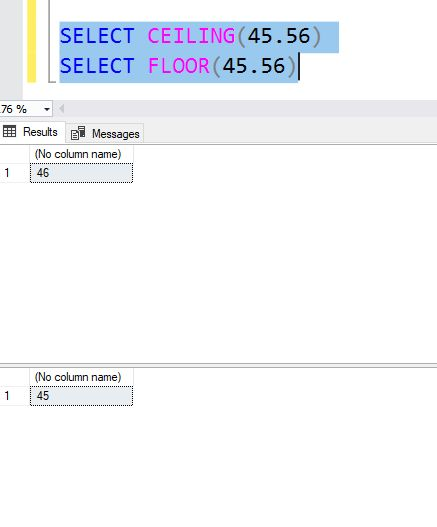My Personal Notes arrow_drop_up